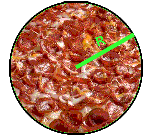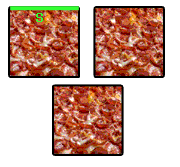From: Kristine

I need help to see if I am doing a problem right.
Which has more area: three small squre pizzas (each piece is 8.5 in.)or one extra-large Pizza (16 in)
For the three small square pizzas I did
A = 8.5 sq X 3 = 216.75 in. sq.
For the large pizza
A = 3.14 X 8 sq = 200.96 in. sq
216.75 - 200.96 = 15.79 in. sq
Three small square pizzas has more area by 15.79 in sq than one large 16 in. pizza

Hi Kristine.

The short answer is that you did it right.

Here are the two situations: three square pieces of pizza, each has side length S = 8.5 inches and one round pizza with diameter 16 inches (that makes the radius R = 8 inches).The area of a square is S2, so the area of the three square pieces is 3S2. The area of a circle is πR2. So when you put your numbers in for R and S, get the numbers and comparison that you already worked out.